# 我想站在你的身旁

## 正文第二章 书店相遇

[更新时间] 2019-08-01 19:20:10 [字数] 2229

“回来啦？”王安的妈妈问道。=&^~\$首发www.zongheng.com%+=-^

“嗯”王安答道。=&^~\$首发www.zongheng.com%+=-^

“在学校怎么样？累么？”=&^~\$首发www.zongheng.com%+=-^

“不累。”=&^~\$首发www.zongheng.com%+=-^

，“你进屋躺一会儿吧，我先把饭拿出来凉一凉。"=&^~\$首发www.zongheng.com%+=-^

“好。”=&^~\$首发www.zongheng.com%+=-^

“饭凉了过来吃吧。”她轻轻说道。=&^~\$首发www.zongheng.com%+=-^

“嗯。”=&^~\$首发www.zongheng.com%+=-^

“今天天气怎么样吗？”=&^~\$首发www.zongheng.com%+=-^

“还行，有点热。”王安嘴里一边嚼着饭菜一边说着。=&^~\$首发www.zongheng.com%+=-^

“那下午就把外套脱了穿短袖吧。”=&^~\$首发www.zongheng.com%+=-^

“嗯，知道了，我饭吃完了去睡觉了。”=&^~\$首发www.zongheng.com%+=-^

“行，去吧。”=&^~\$首发www.zongheng.com%+=-^

“儿子？”=&^~\$首发www.zongheng.com%+=-^

“嗯……”王安坐在床上用一种刚睡醒的人所特有的有气无力的语气答道=&^~\$首发www.zongheng.com%+=-^

“对了你走时候别忘了把门儿锁好。”=&^~\$首发www.zongheng.com%+=-^

“知道了，放心吧。”=&^~\$首发www.zongheng.com%+=-^

“那妈走了？”=&^~\$首发www.zongheng.com%+=-^

“嗯，路上小心点儿啊。”=&^~\$首发www.zongheng.com%+=-^

“王安。”=&^~\$首发www.zongheng.com%+=-^

“啊？啊。”王安忽然有些紧张。=&^~\$首发www.zongheng.com%+=-^

“你没事儿吧？”=&^~\$首发www.zongheng.com%+=-^

“啊，没事儿没事儿，那个，你……你也来买书啊？”=&^~\$首发www.zongheng.com%+=-^

“嗯。”=&^~\$首发www.zongheng.com%+=-^

“啊。”=&^~\$首发www.zongheng.com%+=-^

……=&^~\$首发www.zongheng.com%+=-^

“你直接回家么？”王安尽量让自己看起来不紧张，不过很明显效果不是很好，他已经感觉到自己说话声音都变了。=&^~\$首发www.zongheng.com%+=-^

“嗯。”=&^~\$首发www.zongheng.com%+=-^

“啊，那……再见。”=&^~\$首发www.zongheng.com%+=-^

”再见。”=&^~\$首发www.zongheng.com%+=-^

(快捷键：←)上一章 回目录(快捷键：回车) 下一章(快捷键：→)

### 发表评论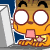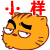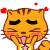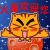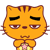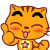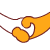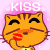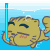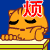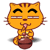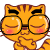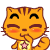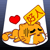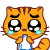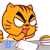12